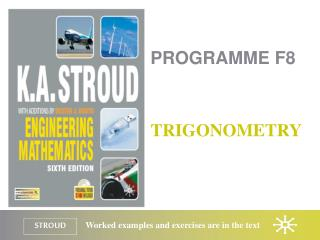Download PresentationPROGRAMME F8# PROGRAMME F8 - PowerPoint PPT Presentation

Download Presentation##### PROGRAMME F8

Download Policy: Content on the Website is provided to you AS IS for your information and personal use and may not be sold / licensed / shared on other websites without getting consent from its author. While downloading, if for some reason you are not able to download a presentation, the publisher may have deleted the file from their server.

- - - - - - - - - - - - - - - - - - - - - - - - - - - E N D - - - - - - - - - - - - - - - - - - - - - - - - - - -
##### Presentation Transcript

1. PROGRAMME F8 TRIGONOMETRY

2. Programme F8: Trigonometry Angles Trigonometric identities Trigonometric formulas

3. Programme F8: Trigonometry Angles Trigonometric identities Trigonometric formulas

4. Programme F8: Trigonometry Angles Rotation Radians Triangles Trigonometric ratios Reciprocal ratios Pythagoras’ theorem Special triangles

5. Programme F8: Trigonometry Angles Rotation When a straight line is rotated about a point it sweeps out an angle that can be measured in degrees or radians A straight line rotating through a full angle and returning to its starting point is said to have rotated through 360 degrees – 360o One degree = 60 minutes and one minute = 60 seconds

6. Programme F8: Trigonometry Angles Radians When a straight line of length r is rotated about one end so that the other end describes an arc of length r the line is said to have rotated through 1 radian – 1 rad

7. Programme F8: Trigonometry Angles Triangles All triangles possess shape and size. The shape of a triangle is governed by the three angles and the size by the lengths of the three sides

8. Programme F8: Trigonometry Angles Trigonometric ratios

9. Programme F8: Trigonometry Angles Trigonometric ratios

10. Programme F8: Trigonometry Angles Reciprocal ratios

11. Programme F8: Trigonometry Angles Pythagoras’ theorem The square on the hypotenuse of a right-angled triangle is equal to the sum of the squares on the other two sides

12. Programme F8: Trigonometry Angles Special triangles Right-angled isosceles

13. Programme F8: Trigonometry Angles Special triangles Half equilateral

14. Programme F8: Trigonometry Angles Special triangles Half equilateral

15. Programme F8: Trigonometry Angles Trigonometric identities Trigonometric formulas

16. Programme F8: Trigonometry Trigonometric identities The fundamental identity Two more identities Identities for compound angles

17. Programme F8: Trigonometry Trigonometric identities The fundamental identity The fundamental trigonometric identity is derived from Pythagoras’ theorem

18. Programme F8: Trigonometry Trigonometric identities Two more identities Dividing the fundamental identity by cos2

19. Programme F8: Trigonometry Trigonometric identities Two more identities Dividing the fundamental identity by sin2

20. Programme F8: Trigonometry Angles Trigonometric identities Trigonometric formulas

21. Programme F8: Trigonometry Trigonometric formulas Sums and differences of angles Double angles Sums and differences of ratios Products of ratios

22. Programme F8: Trigonometry Trigonometric formulas Sums and differences of angles

23. Programme F8: Trigonometry Trigonometric formulas Double angles

24. Programme F8: Trigonometry Trigonometric formulas Sums and differences of ratios

25. Programme F8: Trigonometry Trigonometric formulas Products of ratios

26. Programme F8: Trigonometry Learning outcomes • Convert angles measured in degrees, minutes and seconds into decimal degrees • Convert degrees into radians and vice versa • Use a calculator to determine the values of trigonometric ratios for any acute angle • Verify trigonometric identities